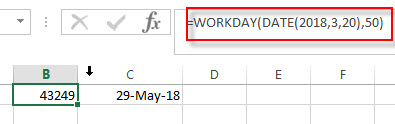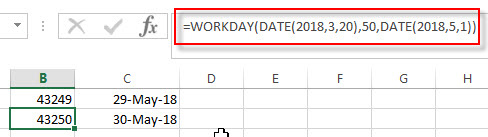# Excel WORKDAY Function

This post will guide you how to use Excel WORKDAY function with syntax and examples in Microsoft excel.

### Description

The Excel WORKDAY function returns a serial number that represents a date that is the indicated number of working days before or after the starting date you specified. So you can add a specified number of working days to the starting date and then returns a serial date. The working days will exclude weekends and any dates specified as holidays.

You can use the WORKDAY function to calculate working days and non-working days.

The WORKDAY function is a build-in function in Microsoft Excel and it is categorized as a DATE and TIME Function.

The WORKDAY function is available in Excel 2016, Excel 2013, Excel 2010, Excel 2007, Excel 2003, Excel XP, Excel 2000, Excel 2011 for Mac.

### Syntax

The syntax of the WORKDAY function is as below:

`=WORKDAY(start_date, days, [holidays])`

Where the WORKDAY function arguments is:

• Start_date –This is a required argument. The starting date from which you want to count the number of working days. The date should be typed as a valid Excel time a serial date.
• days – This is a required argument. The number of working days that you want to add. A positive value for days yields a future date; a negative value yields a past date.
• holidays – This is an optional argument. The list of holidays that you want to exclude from the working days. It can be a range of cells that contain the holiday dates or it can be a list of serial numbers that represent the holiday dates.

Note:

• If any argument is not a valid Excel date, a #VALUE! Error is returned.
• If start date value plus days is an invalid date, the WORKDAY function returns #NUM! Error.
• A serial date is how the Microsoft Excel stores dates and it represents the number of days since 1900-01-01, so the January 1, 1900 date is serial number 1 by default.
• If days value is not a integer number, it will be truncated.

### Excel WORKDAY Function Examples

The below examples will show you how to use Excel WORKDAY Function to return the working days from the start date.

1# get the date 50 workdays from the starting date “3/20/2018”, enter the following formula in Cell B1.

`=WORKDAY(DATE(2018,3,20),50)`2# get the date 50 workdays from the starting date “3/20/2018”, excluding holidays 5/1/2018, type the following formula in Cell B2.

`=WORKDAY(DATE(2018,3,20),50,DATE(2018,5,1))`### Related Functions

• Excel WEEKDAY function
The Excel WEEKDAY function returns a integer value representing the day fo the week for a given Excel date and the value is range from 1 to 7.The syntax of the WEEKDAY function is as below:=WEEKDAY (serial_number,[return_type])…
• Excel DAY function
The Excel DAY function returns a day of a date (from 1 to 31).The DAY function is a build-in function in Microsoft Excel and it is categorized as a DATE and TIME Function.The syntax of the DAY function is as below:= DAY (date_value)…
• Excel DATE function
The Excel DATE function returns the serial number for a date.The syntax of the DATE function is as below:= DATE (year, month, day)…

### More Excel Workday Function Examples

• Calculating Future Date
If you want to calculate a new future date excluding weekends, and you need to use the WORKDAY function to create a new formula to exclude all weekends in the future date.…